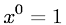Equations > Algebra > Exponents/Powers > Exponent Equal to Zero Rule

### Exponent Equal to Zero RuleLatex Code:

MathML Code:

 ${x}^{0}=1$

MathType 5.0: